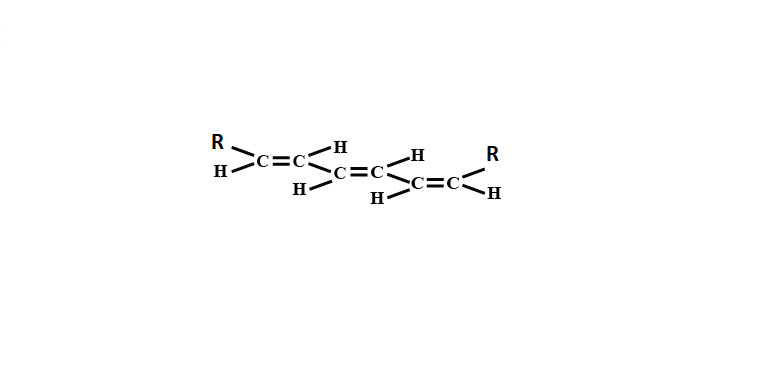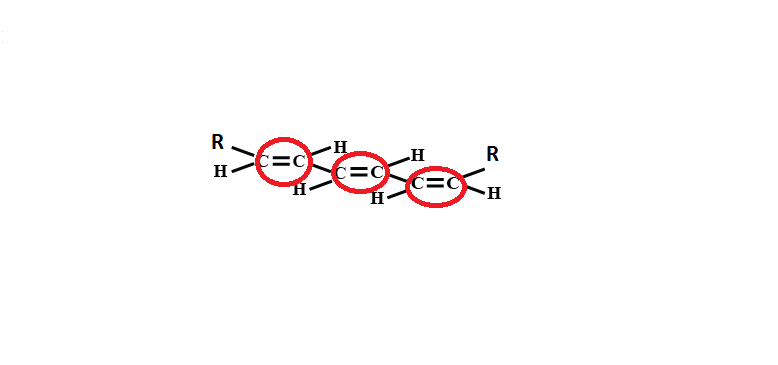QuestionAnswers

# Number of geometrical isomers for the molecule(A).2(B).4(C).6(D).8Verified
91.5k+ views
Hint: To write the geometrical isomerism we have to identify the bonding connectivity between atoms. The molecules having the same bonding connectivity can be a geometrical isomer.

The stereoisomers are defined as the molecules have the same bonding connectivity and different molecular configuration. Stereoisomers are of two type’s i.e. optical and geometrical isomers.
Geometrical isomers are the isomers which differ by the arrangement along the double bond ring and other rigid structure.
Given in the above molecule:
3 double bonds which are shown below and the molecule is symmetric:The total number of geometrical isomers will be= ${{2}^{n-1}}+{{2}^{\dfrac{n-1}{2}}}$
Put the value of n =3
${{2}^{3-1}}+{{2}^{\dfrac{3-1}{2}}}$
${{2}^{2}}-{{2}^{1}}$
$4+2=6$
Hence the number of geometrical isomers for the molecule is 6.

So, Correct answer is option (C).